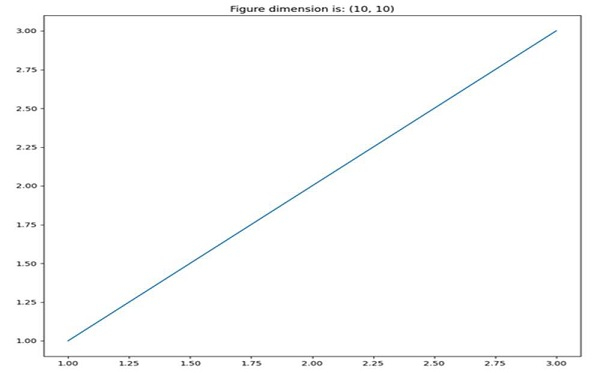# Change figure size and figure format in Matplotlib

Using the figsize attribute of figure(), we can change the figure size. To change the format of a figure, we can use the savefig method.

## Steps

• Store the figure size in the variable.

• Create a new figure, or activate an existing figure, with given figure size.

• Plot the line using x.

• Set the image title with its size.

• Save the figure using savefig() method.

## Example

from matplotlib import pyplot as plt

figure_size = (10, 10)
plt.figure(figsize=figure_size)
x = [1, 2, 3]
plt.plot(x, x)
plt.title("Figure dimension is: {}".format(figure_size))
plt.savefig("imgae.png", format="png")

## Output